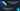# Dart Programming Language Tutorial Part 7 | If Else and Ternary Operators in Dart

September 10th, 2018
|

In this post we will cover the if-else statement in Dart. If-else block is one of the basic building blocks of many programming languages, let's look into it right away.

# Conditions in a Program

Many a times in a program we are required to check for a particular condition. Based on the truth of condition i.e. if the condition is either is true or false we execute a set of instructions. In Dart `If-Else` is one of the few ways to check for conditions in our program. On it's own, if-else is pretty simple to write and understand, it really shines when it is combined with other powerful concepts of the language and can be used to write some pretty complex logic. Let's look into if-else syntax and some examples.

# If-Else in Dart

The syntax of an if-else statement is very simple. Let's first start with only an if statement.

``````    if(condition){
// code to execute when condition is true
}``````

So above is a syntax of a simple if block. We write the condition in the paranthesis and if the condition evaluates to `true` the code inside the `{}` will be run. Pretty simple ha..!!!. Now Let's look at the syntax of an if-else block.

``````    if(condition){
// run when condition is true
}else{
// run when condition is false
}``````

Now we have a `if-else` syntax here. We condition in the paranthesis as before and if the condition evaluates to `true` the code inside the if block runs however if some unfortunate reason the condition evaluates to false, the code inside `else` block runs. We can also combine multiple if-else blocks to create a chain of `if-elseif-else` block. Let's look at some examples.

``````    var a = 3;

if(a<4){
print("condition is true");   // prints this line
}else{
print("condition is false");    // skips this line
}

var b = 5;

if(b<4){
print("condition is true");  // skips this line
}else{
print("condition is false");    // prints this line
}``````

Here we have two examples, in one of them the condition is true, while the condition evaluates to false in the other one. In the case where condition evaluates to true, print in the if block is run, while in case where condition evaluates to false, print in the else block is run. Let's look at another example where we can chain multiple if-else.

``````    // marks 80-100 A+
// marks 60-80 A
// marks 40-60 B
// marks < 40 C

var marks = 90;

if ( marks > 80 && marks <= 100 ){
print("A+");  // Prints A+
}else if( marks >60 && marks <= 80){
print("A");
}else if(marks > 40 && marks <= 60){
print("B");
}else{
print("C");
}``````

Another example where we demonstrate chaining of `if-else` . Here the first if block's condition evaluates to true so the print is run and rest of code is skipped. Now let's move on to ternary operators.

# Ternary Operators in Dart

Ternary operators are nothing but another way to write an if-else block in a single line. It is well-and-good if you want to write quick and small conditions but we shouldn't use this for complex logical checks as this might get messy. Let's look into the syntax.

``````    var a = 3;

print((a<4) ? "condition is true" : "condition is false" );``````

Okay so everything we have inside the print is part of our ternary operator. Ternary operators start with a condition, usually it's good to wrap ur condition in a paranthesis , but it is not required. Next we have a `?`. Anything we write between `?` and `:` is run if the condition is true, and anything we write after the `:` is run id the condition was false. In our case since the condition was true, it will return `"condtion is true"` and that would be printed. This is it for this one. Will see you in the next one.